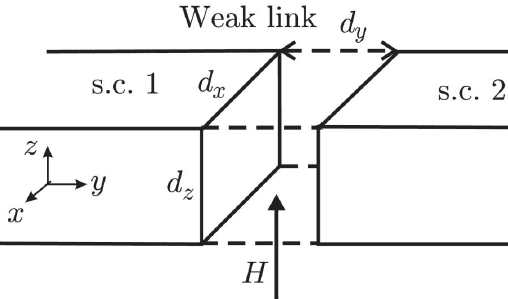# Total Josephson current through junction with magnetic field

• Mr_Allod

#### Mr_Allod

Homework Statement
Show that the total Josephson current though a Josephson Junction is:
$$d_xd_yj_0 \frac {\phi_0}{2\pi \phi} \left[ \cos(\psi_0) - \cos(\psi_0 + \frac{2\pi \phi}{\phi_0}) \right]$$
Relevant Equations
Total flux through the junction: ##\phi = Bd_xd_y##
Current density at position x: ##J(x) = j_0\sin(\psi_0 + \frac{2\pi x}{\phi_0}Bd_y)##
Hello there,

I am given a diagram of a Josephson Junction like so:With a magnetic field ##B = \mu_oH## in the z-direction. I'm reasonably sure ##d_x,d_y,d_z## are normal lengths, not infinitesimal lengths although that is up for debate. Using the above equations I rearrange the expression for J(x) to be in terms of ##\phi## and compute the following integral:

$$\int_{\frac {-d_x}{2}}^{\frac {d_x}{2}}\int_{\frac {-d_y}{2}}^{\frac {d_y}{2}} j_0\sin(\psi_0 + \frac{2\pi \phi}{\phi_0d_x}x)d_xd_y$$

This yielded:

$$d_xd_yj_0 \frac {\phi_0}{2\pi \phi} \left[ \cos(\psi_0 - \frac{\pi \phi}{\phi_0}) - \cos(\psi_0 + \frac{\pi \phi}{\phi_0}) \right]$$

Where ##\psi_0## is the phase difference at ##x=0##. This is not the same as the expression we were given in the problem but it is quite similar which makes me think I am on the right track. Is there something else I need to do with the expression we are given before integrating to find the total current?

$$\int_{\frac {-d_x}{2}}^{\frac {d_x}{2}}\int_{\frac {-d_y}{2}}^{\frac {d_y}{2}} j_0\sin(\psi_0 + \frac{2\pi \phi}{\phi_0d_x}x)d_xd_y$$

Shouldn't the integration be over ##x## and ##z## rather than ##x## and ##y##?

Maybe the origin of the coordinate system is such that ##x## ranges from ##0## to ##d_x## rather than from ##-d_x/2## to ##d_x/2## and ##z## ranges from ##0## to ##d_z## rather than from ##-d_z/2## to ##d_z/2##.

Shouldn't the integration be over ##x## and ##z## rather than ##x## and ##y##?

Maybe the origin of the coordinate system is such that ##x## ranges from ##0## to ##d_x## rather than from ##-d_x/2## to ##d_x/2## and ##z## ranges from ##0## to ##d_z## rather than from ##-d_z/2## to ##d_z/2##.
I think you're right on both counts. I suppose if I had paid more attention to the figure I would have realized I'm using the wrong origin. Integrating from ##0## to ##d_{x,z}## gives the right answer. With regards to the area of integration, I think I was just forcing the solution to be in line with the expression we're given and it's entirely possible there was a typo in it. It wouldn't be the first time.

•TSny